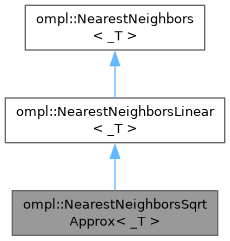ompl::NearestNeighborsSqrtApprox< _T > Class Template Reference

A nearest neighbors datastructure that uses linear search. The linear search is done over sqrt(n) elements only. (Every sqrt(n) elements are skipped). More...

#include <ompl/datastructures/NearestNeighborsSqrtApprox.h>

Inheritance diagram for ompl::NearestNeighborsSqrtApprox< _T >:[legend]

## Public Member Functions

void clear () override
Clear the datastructure.

void add (const _T &data) override
Add an element to the datastructure.

void add (const std::vector< _T > &data) override
Add a vector of points.

bool remove (const _T &data) override
Remove an element from the datastructure.

_T nearest (const _T &data) const override
Get the nearest neighbor of a point.Public Member Functions inherited from ompl::NearestNeighborsLinear< _T >
bool reportsSortedResults () const override
Return true if the solutions reported by this data structure are sorted, when calling nearestK / nearestR.

void nearestK (const _T &data, std::size_t k, std::vector< _T > &nbh) const override
Return the k nearest neighbors in sorted order.

void nearestR (const _T &data, double radius, std::vector< _T > &nbh) const override
Return the nearest neighbors within distance radius in sorted order.

std::size_t size () const override
Get the number of elements in the datastructure.

void list (std::vector< _T > &data) const override
Get all the elements in the datastructure.Public Member Functions inherited from ompl::NearestNeighbors< _T >
virtual void setDistanceFunction (const DistanceFunction &distFun)
Set the distance function to use.

const DistanceFunctiongetDistanceFunction () const
Get the distance function used.

## Protected Member Functions

void updateCheckCount ()
The maximum number of checks to perform when searching for a nearest neighbor.

## Protected Attributes

std::size_t checks_ {0}
The number of checks to be performed when looking for a nearest neighbor.

std::size_t offset_ {0}
The offset to start checking at (between 0 and checks_)Protected Attributes inherited from ompl::NearestNeighborsLinear< _T >
std::vector< _T > data_
The data elements stored in this structure.Protected Attributes inherited from ompl::NearestNeighbors< _T >
DistanceFunction distFun_
The used distance function.

## Additional Inherited MembersPublic Types inherited from ompl::NearestNeighbors< _T >
using DistanceFunction = std::function< double(const _T &, const _T &)>
The definition of a distance function.

## Detailed Description

### template<typename _T> class ompl::NearestNeighborsSqrtApprox< _T >

A nearest neighbors datastructure that uses linear search. The linear search is done over sqrt(n) elements only. (Every sqrt(n) elements are skipped).

• Search for nearest neighbor is O(sqrt(n)).
• Search for k-nearest neighbors is O(n log(k)).
• Search for neighbors within a range is O(n log(n)).
• Adding an element to the datastructure is O(1).
• Removing an element from the datastructure O(n).

Definition at line 57 of file NearestNeighborsSqrtApprox.h.

The documentation for this class was generated from the following file: